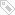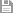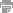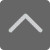## 爱智康资讯站

### 课程咨询: 4000-121-121

#### 学习规划

TOP
##### 申请学习规划# 【苏科版】南京七年级上册数学书习题3.3答案

2019-07-12 10:54:58 　来源：爱智康

【智康语】【苏科版】南京七年级上册数学课书习题3.3答案！课后习题是针对当堂课的一次巩固和总结的练习，需要同学们自行解答。今天南京爱智康小编为大家整理了【苏科版】南京七年级上册数学书习题3.3答案，供大家参考、学习！

（1）2a+2b

=2×2+2×3

=4+6

=10

（2）2（a+b）

=2×（2+3）

=2×5

=10

（3）a2b2

=22×32

=4×9

=36

（4）（ab）2

=（2×3）2

=62

=36

（5）a2+b2

=22+32

=4+9

=13

（6）（a+b）2

=（2+3）2

=52

=25

（7）a2+2ab+b2

=22+2×2×3+32

=4+12+9

=25

（8）2（2a-b）3-（2a-b）2+8（2a-b）

=2×（2×2-3）3-（2×2-3）2+8×（2×2-3）

=2×1-1+8×1

=2-1+8

=9

tC=5/9×（64.4-32）=5/9×32.4=18（℃），

②a2-πa2/4

③a2-πa2/4

（2）①100-25π

②100-25π

③100-25π

V球=4/3 πr3

S地球≈5.1×108 （km2 ）

V地球≈1.1×1012 （km3 ）

以上是【苏科版】南京七年级上册数学书习题3.3答案，希望能够帮助到同学们，另外南京爱智康暑期初一入学助力课火热上线中，完美过渡新初一，咨询热线：4000-121-121标签：保存　｜打印　｜关闭